### Home > MC2 > Chapter 6 > Lesson 6.1.3 > Problem6-28

6-28.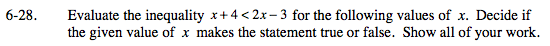Substitute each value for x in the inequality given above.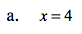4 + 4 < 2(4) − 3

By simplifying, we get:
8 < 8 − 3
8 < 5
Is 8 less than 5?

False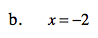−2 + 4 < 2(−2) − 3

By simplifying, we get:
2 < −4 − 3
2 < −7
Is 2 less than -7?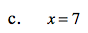See parts (a) and (b).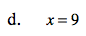See parts (a) and (b).